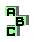ANTIMEDIAL TRIANGLE

 r12 Brocard points, third Brocard point r52 Carnot triangle, Gossard triangle, medial triangle, homothetic triangles, Euler line d retrocenter d Nagel point r97 Kiepert hyperbola, Wallace hyperbola r257 circumtangential triangle, Stammler circles, Morley triangle, homothetic triangles r447 circumcircle, orthocenter, circumcenter, Brocard line r490 orthocenter, point X(264) r530 altitudes, orthocenter, circumcircle r538 Vecten squares, Kiepert hyperbola r540 circumcircle, cevian triangle r1085 anticevian triangle, complement, anticomplement, centroid, inconic, perspector, cevian product r1228 orthic triangle, ninecircle, point X(115) r1240 orthocenter, Euler line r1339 circumcenter, cevian triangle, circumcircle, homothetic triangles r1404 Neuberg triangle, perspective, medial triangle, Wallace hyperbola r1405 Neuberg triangle, orthologic triangles, cevian quotient, centroid, circumcenter, symmedian point r1406 Neuberg triangle, perspective, point X(262) r1668 centroid, tripolar, Steiner circumellipse, cevian triangle r1693 incircle, tangent circles r1865 ninecenter, perspective, orthic triangle, Carnot triangle, point X(110), point X(52) r2011 intouch triangle, Euler line, incircle, Feuerbach point, point X(80)